Home > A2C > Chapter 9 > Lesson 9.2.3 > Problem9-106

9-106.
1. Make a sketch of a graph p(x) so that p(x) = 0 would have the indicated number and type of solutions. Homework Help ✎

1. 5 real solutions

2. 3 real and 2 complex

3. 4 complex

4. 4 complex and 2 real

5. For parts (a) through (d), what is the lowest degree each function could have?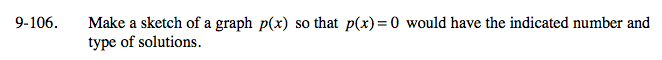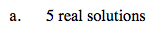Drag the polynomial function to see where it intersects the x-axis.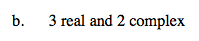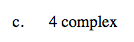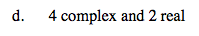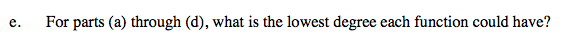The degree needs to be at least as high as the number of solutions.

Use the eTool below to explore the problem further.
Click the link at right for the full version of the eTool: A2C 9-106 HW eTool